### Home > CALC > Chapter 10 > Lesson 10.1.5 > Problem10-43

10-43.
1. Examine the following series. Use one of the tests you have learned so far to determine if the series converges or diverges. Name the test that you used. Homework Help ✎

1.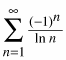2.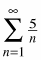3.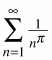4.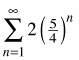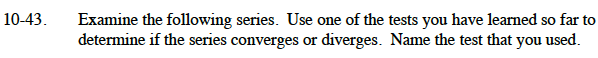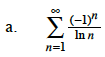This is an alternating series.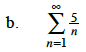This is a form of the harmonic series.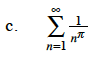π is just a number, so this is a p-Series.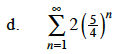This is a geometric series. What is the value of r?